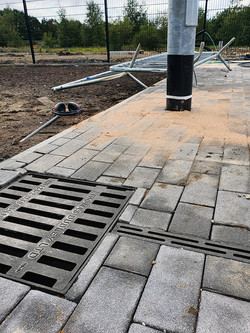###### Request your FREE copy of AquaCALC here:

Thanks for submitting!##### ​

When sizing Swarm Aqua® channels at Swarm, AquaCalc™ models the conduit as spherical, open pipework with the flow originating from a single point at the high end of the channel. This then removes the need to factor in turbulence

from linear interception and provides a ‘worst-case scenario’ of flow through the channel.

When calculating surface runoff (or the volume of water the channel must cater for), we multiply the rainfall rate in l/s/m (often translated from mm/hr) by the catchment area in m2, and the permeability factor of the surface finish in question. The following permeability factors are used:

Concrete: 100%
Asphalt: 85%
Paving: 60%

The result is a required flow rate, denoted commonly as ‘Q’ that the channel must be sized sufficiently to cater for.

When we size channels at Swarm, the ‘Q’ value is then increased by 10% as a factor of safety and used as the benchmark to check which channel configurations will provide sufficient volume for the given situation.

From the length of the channel, an 'if loop' is carried out to test the capacities of Swarm Aqua® channels until the smallest working channel is found.

The standard flow calculation formula of Q=AV where

A = the area of the pipe and

V = the velocity of the water is used.

###### Manning’s Equation

One the most commonly used equations governing Open Channel Flow is known as the Mannings’s Equation. The Manning’s equation is an empirical equation that applies to uniform flow in open channels and is a function of the channel velocity, flow area and channel slope.

Manning’s equation is applied to the Swarm Aqua® channels in order to populate the achievable flow rates within each channel breakdown in the matrix. In addition to the simple flow calculation attributed to the water flowing through the conduit, an average achieved gradient is also applied when using a stepped fall calculation. This is applied as the differential between high and low invert values of the conduit, divided by the length of the run. This average gradient then has a positive effect on the flow of water through the conduit.

##### ​